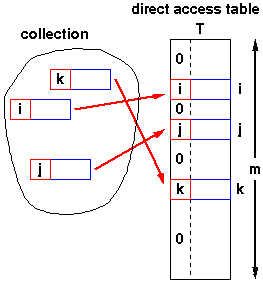# Direct Address Table

Direct Address Table is a data structure that uses arrays to store the data. In the Direct Address Table, the element key values are used as their indexes in the array to store the record by the technique known as Direct Addressing.

So now, the question arises what is Direct Addressing?

It is a technique in which we make use of direct address tables to map their values with their keys.

It uses the keys as indexes of the buckets and then stores the values at those bucket locations.

Though Direct Addressing does facilitate fast searching, fast inserting, and fast deletion operations, at a cost, Direct Address Table cannot handle collisions.

If a different data for the same key needs to be inserted in the table, then it won’t happen here.

Therefore, DAT cannot be used in the real world practically, and this function of DAT makes it different from Hash Tables.

Hash Tables overcome the major disadvantage of DAT by resolving hashing collisions by using hash function through various hashing techniques such as:

• Linear Probing
• Chaining

Now, what is Hash Function?

A hash function is a function that can be used to map data of arbitrary size to fixed-sized values. The values returned by hash functions are called hash values.

Hash functions should be chosen carefully because it minimizes the total number of collisions as much as possible.

Now coming back to the process of Direct Address Table:

Let’s take an example,

We have a problem searching for an element with a value of 15 in the range of [0,100000].

Now using binary search we can solve this problem in O(Nlogn) time complexity, where N is used for the iteration process, but with DAT we can solve this problem in O(N) where N is for the iteration process, searching will take O(1) time complexity.

Create an array of size (100000 + 1) and initialize every record with value 0.

Now iterate over the array, and update the record with “1” for every element encountered.

After this step check if DAT is equal to 0 or not.

If it is then, 15 is present else 15 is not present.### Algorithm of DAT functions

SEARCH (T, K) // O(1) operation

return T[k];

INSERT (T, x) // O(1) operation

T[x.key] = x;

DELETE (T, x) // O(1) operation

T[x.key] = NIL;